NEET  >  Bohr's Theory Of Hydrogen Atom

# Bohr's Theory Of Hydrogen Atom

Test Description

## 19 Questions MCQ Test Chemistry Class 11 | Bohr's Theory Of Hydrogen Atom

Bohr's Theory Of Hydrogen Atom for NEET 2023 is part of Chemistry Class 11 preparation. The Bohr's Theory Of Hydrogen Atom questions and answers have been prepared according to the NEET exam syllabus.The Bohr's Theory Of Hydrogen Atom MCQs are made for NEET 2023 Exam. Find important definitions, questions, notes, meanings, examples, exercises, MCQs and online tests for Bohr's Theory Of Hydrogen Atom below.
Solutions of Bohr's Theory Of Hydrogen Atom questions in English are available as part of our Chemistry Class 11 for NEET & Bohr's Theory Of Hydrogen Atom solutions in Hindi for Chemistry Class 11 course. Download more important topics, notes, lectures and mock test series for NEET Exam by signing up for free. Attempt Bohr's Theory Of Hydrogen Atom | 19 questions in 19 minutes | Mock test for NEET preparation | Free important questions MCQ to study Chemistry Class 11 for NEET Exam | Download free PDF with solutions
 1 Crore+ students have signed up on EduRev. Have you?
Bohr's Theory Of Hydrogen Atom - Question 1

### Which of the following is correct?

Detailed Solution for Bohr's Theory Of Hydrogen Atom - Question 1

Because isotones means the same number of neutron, So, from the question, option c is right, number of neutron in k is,39-19= 20,and,the number of neutron in ca is also 40-20=20 so it is isotones.

Bohr's Theory Of Hydrogen Atom - Question 2

### ‘Hartree’ is the atomic unit of energy and is equal to

Detailed Solution for Bohr's Theory Of Hydrogen Atom - Question 2

The potential energy of an electron in the first Bohr’s orbit in the H-atom is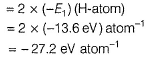This energy is defined as an atomic unit of energy called HARTREE.

Bohr's Theory Of Hydrogen Atom - Question 3

### Wave number of a spectral line for a given transition is x cm-1 for He+, then its value for Be3+ (isoelectronic of He+)for the same transition is

Detailed Solution for Bohr's Theory Of Hydrogen Atom - Question 3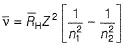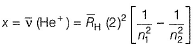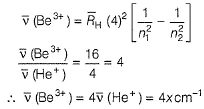Bohr's Theory Of Hydrogen Atom - Question 4

Which of the following electronic transitions requires that the greatest quantity of energy be absorbed by a hydrogen atom ?

Detailed Solution for Bohr's Theory Of Hydrogen Atom - Question 4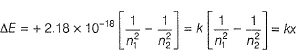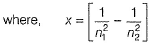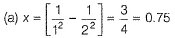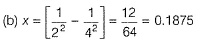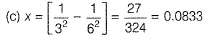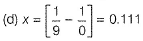Therefore, electronic transition (a) requires greatest quantity of energy.

Bohr's Theory Of Hydrogen Atom - Question 5

An electron in H-atom in its ground state absorbs 1.5 times as much as energy as the minimum required for its escape from the atom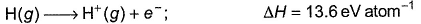Q.

Thus, kinetic energy given to the emitted electron is

Detailed Solution for Bohr's Theory Of Hydrogen Atom - Question 5

E1 = Energy of H-atom in the ground state = 13.6 eV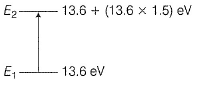Energy absorbed = (13.6 x 1.5) = 20.4 eV
E2 = Energy of the excited state
= 13.6+ 20.4= 34.0 eV
ΔE = KE = (E2 - E1)
= 34.0 - 13.6 = 20.4 eV

Bohr's Theory Of Hydrogen Atom - Question 6

Ionisation energy of He+ is 19.6x10-18 J atom -1. The energy of the first stationary state (n = 1)of Li2+ is

Detailed Solution for Bohr's Theory Of Hydrogen Atom - Question 6

Ionisation energy = - Energy of the electron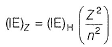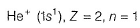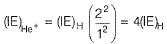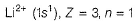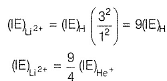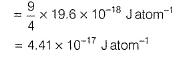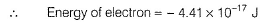Bohr's Theory Of Hydrogen Atom - Question 7

The kinetic energy of an electron in the second Bohr orbit of a hydrogen atom is (a0 is Bohr radius)

[AIEEE 2012]

Detailed Solution for Bohr's Theory Of Hydrogen Atom - Question 7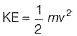Also, angular momentum is quantised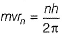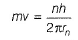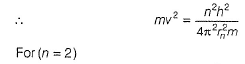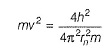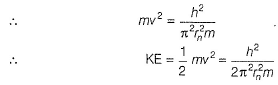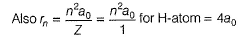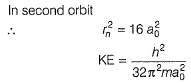Bohr's Theory Of Hydrogen Atom - Question 8

Energy of the electron in nth orbit is given by E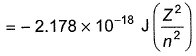Wavelength of light required to excite an electron in an H-atom from level n = 1 to n = 2 will be (h = 6.62 x 10-34 J s ; c = 3.0 x 108ms -1)

[AIEEE 2012]

Detailed Solution for Bohr's Theory Of Hydrogen Atom - Question 8

For H - atom , Z = 1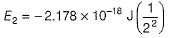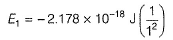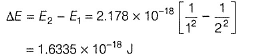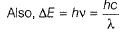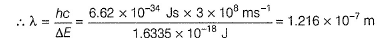Bohr's Theory Of Hydrogen Atom - Question 9

The potential energy of an electron in the second Bohr's orbit of the he±

Detailed Solution for Bohr's Theory Of Hydrogen Atom - Question 9

Bohr's Theory Of Hydrogen Atom - Question 10

In Lyman series, shortest wavelength of H-atom appears at x m, then longest wavelength in Balmer series of He+ appear at

Detailed Solution for Bohr's Theory Of Hydrogen Atom - Question 10

For the spectral line in H -atom and H -like species (one electron)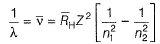For Lyman series
For shortest wavelength (maximum wave number) n2 → ∞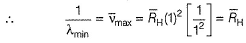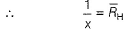For longest wave length (minimum wave number), n2 = (n1, + 1).
For Balmer series, n1 = 2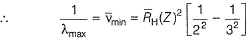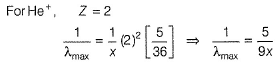Bohr's Theory Of Hydrogen Atom - Question 11

If the radius of the first Bohr orbit is x, then de-Broglie wavelength of the electron in the third orbit is nearly

Detailed Solution for Bohr's Theory Of Hydrogen Atom - Question 11

Angular momentum is quantised , hence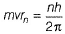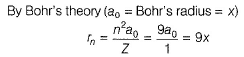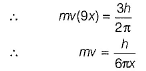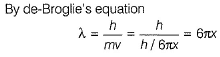Bohr's Theory Of Hydrogen Atom - Question 12

Direction (Q. Nos. 12-13) This section contains a paragraph, wach describing  theory, experiments, data etc. three Questions related to paragraph have been given.Each question have only one correct answer among the four given  ptions  (a),(b),(c),(d)

Radius of Bohr’s orbit of H-atom is 52.9 pm. An emission in H-atom starts from the orbit having radius 1.3225 nm and ends at 211.6 pm.

Q.

Wavelength (in nm) associated with this emission is

Detailed Solution for Bohr's Theory Of Hydrogen Atom - Question 12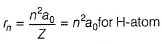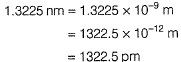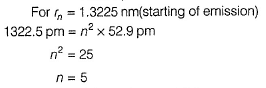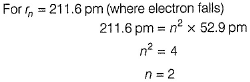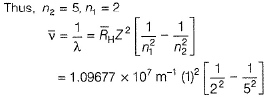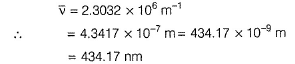Bohr's Theory Of Hydrogen Atom - Question 13

Radius of Bohr’s orbit of H-atom is 52.9 pm. An emission in H-atom starts from the orbit having radius 1.3225 nm and ends at 211.6 pm.

Q.  Spectral line appears in .......... region.

Detailed Solution for Bohr's Theory Of Hydrogen Atom - Question 13

Emission (n2 = 5 to n1 = 2) is called Balmer series and appears in visible region.

Bohr's Theory Of Hydrogen Atom - Question 14

Direction (Q. Nos. 14 and 15) Choice the correct combination of elements and column I and coloumn II are given as option (a), (b), (c) and (d), out of which ONE option is correct.

Q.

Match the equation in Column I with the name type in Column II.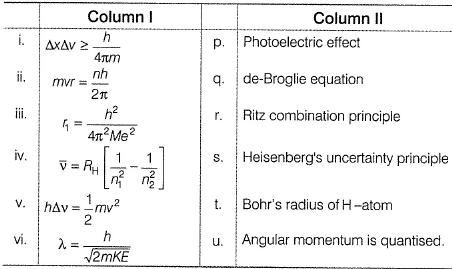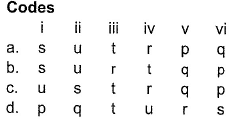Detailed Solution for Bohr's Theory Of Hydrogen Atom - Question 14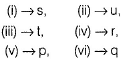Bohr's Theory Of Hydrogen Atom - Question 15

lf En = total energy, Kn = kinetic energy, Vn = potential energy and rn = radius of the nth orbit, then based on Bohr’s theory, match the parameter in Column I with the values in Column II.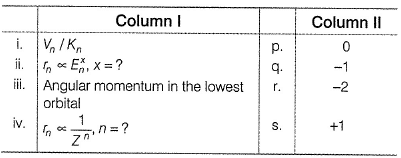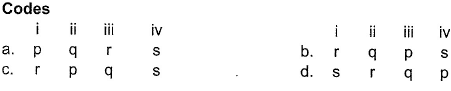Detailed Solution for Bohr's Theory Of Hydrogen Atom - Question 15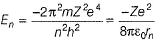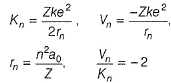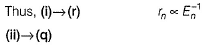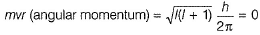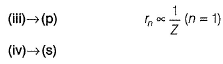*Answer can only contain numeric values
Bohr's Theory Of Hydrogen Atom - Question 16

Direction (Q. Nos. 16 - 19) This section contains 4 questions. when worked out will result in an integer from 0 to 9 (both inclusive)

Q. The energy of an electron in the first Bohr orbit of H-atom is -13.6 eV. What is the possible value of quantum number for the excited state to have energy -3.4 eV?

Detailed Solution for Bohr's Theory Of Hydrogen Atom - Question 16

E = -13.6×Z2/ n
For H atom, Z=1 and given energy = -3.4
So, we have, -3.4 = -13.6/n2
Or n = 2

*Answer can only contain numeric values
Bohr's Theory Of Hydrogen Atom - Question 17

An emission is Be3+ in observed at 2.116 A°. In which orbit is it placed?

Detailed Solution for Bohr's Theory Of Hydrogen Atom - Question 17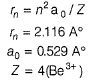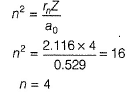*Answer can only contain numeric values
Bohr's Theory Of Hydrogen Atom - Question 18

At what minimum atomic number, a transition from n = 2 to n = 1 energy level results in the emission of X-rays with wavelength 3.0 x 10-8 m?

Detailed Solution for Bohr's Theory Of Hydrogen Atom - Question 18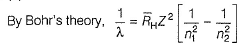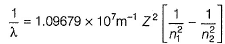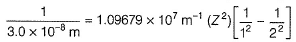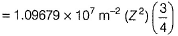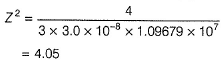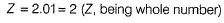*Answer can only contain numeric values
Bohr's Theory Of Hydrogen Atom - Question 19

Find the number of waves made by a Bohr’s electron in one complete revolution in its 3rd orbit

Detailed Solution for Bohr's Theory Of Hydrogen Atom - Question 19

circumference of the orbit = 2πrn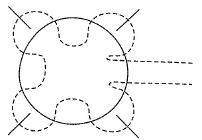Number of waves in one complete revolution = nλ
For third orbit = nλ = 3λ
Thus, three waves are formed in one revolution.

## Chemistry Class 11

199 videos|330 docs|229 tests
Information about Bohr's Theory Of Hydrogen Atom Page
In this test you can find the Exam questions for Bohr's Theory Of Hydrogen Atom solved & explained in the simplest way possible. Besides giving Questions and answers for Bohr's Theory Of Hydrogen Atom, EduRev gives you an ample number of Online tests for practice

## Chemistry Class 11

199 videos|330 docs|229 tests

### How to Prepare for NEET

Read our guide to prepare for NEET which is created by Toppers & the best Teachers(Scan QR code)